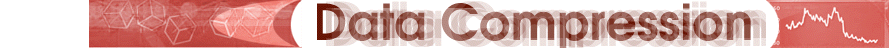The Concept
The Algorithm

- Layer Coding Options
- Hybrid Filter Bank
- Psychoacoustics Model
- Bit Allocation
Bit Allocation

Through an iterative algorithm, the bit allocation uses information from the psychoacoustic model to determine the number of code bits to be allocated to each subband. This process can be described using the following formula:

MNRdB = SNRdB - SMRdB

 where: MNRdB is the mask-to-noise ratio SNRdB is the signal-to-noise ratio, given with the MPEG audio standard in a table SMRdB is the signal-to-mask ratio, derived from the psychoacoustic model

Then the subbands are placed in order of lowest to highest mask-to-noise ratio, and the lowest subband is allocated the smallest number of code bits and this process continues until no more code bits can be allocated (Pan 5).

Two nested iteration loops called the rate loop and the distortion loop serve to quantize and code in MP3 encoders. The quantized values are coded using Huffman methods, which is lossless.